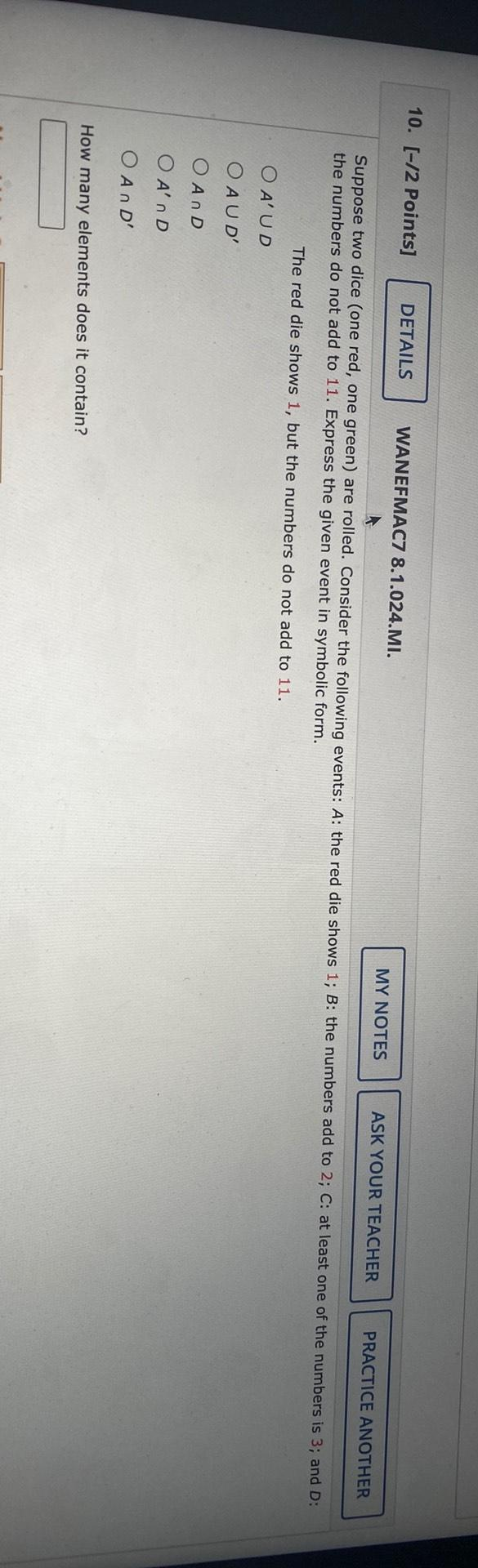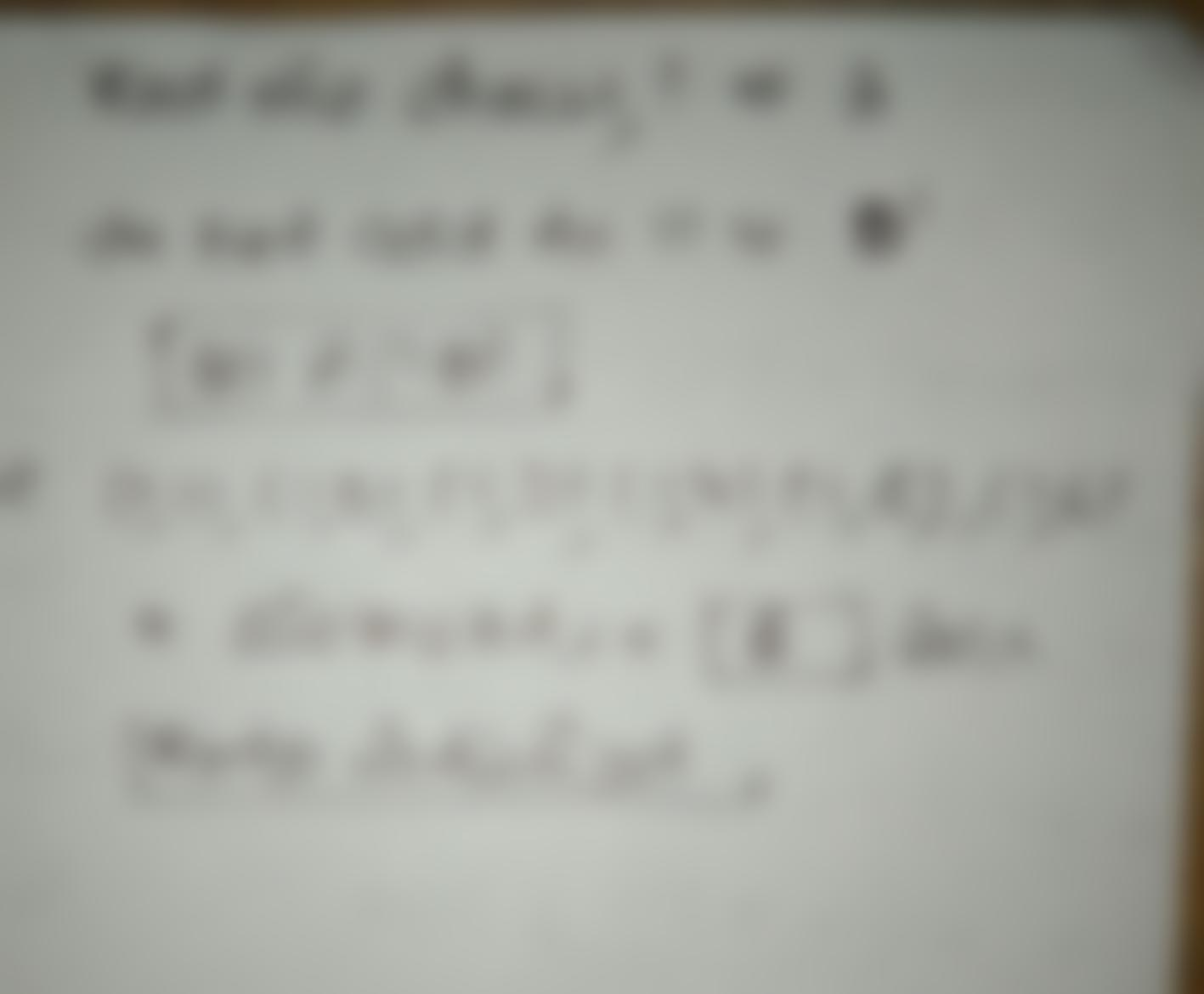Question:

10. [-12 Points] DETAILS WANEFMAC7 8.1.024.MI. MY NOTES ASK YOUR TEACHER PRACTICE ANOTHER Suppose two dice (one red, one green)10. [-12 Points] DETAILS WANEFMAC7 8.1.024.MI. MY NOTES ASK YOUR TEACHER PRACTICE ANOTHER Suppose two dice (one red, one green) are rolled. Consider the following events: A: the red die shows 1; B: the numbers add to 2; C: at least one of the numbers is 3; and D: the numbers do not add to 11. Express the given event in symbolic form. The red die shows 1, but the numbers do not add to 11. O AUD AUD O AND O AD O AND How many elements does it contain?